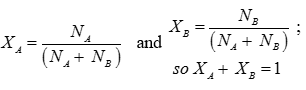# Chemistry Solutions - Online Test

Q1. Two solutions have different osmotic pressures. The solution of higher osmotic pressure is called
Explaination / Solution:

Hypertonic solutions are having high Osmotic Pressure.

Q2. At constant temperature the osmotic pressure of a solution is
Explaination / Solution:

Л=cRT

Q3. The sum of all mole fraction for a mixture is always equal to
Explaination / Solution:Q4. The rate at which a solid dissolves in liquid does not depend on
Explaination / Solution:

The rate at which a solid dissolves in liquid does not depend on pressure.

Q5. Solubility of gas decreases with
Explaination / Solution:

Because of increase in kinetic energy of gas molecules with temperature there tendency to escape from the liquid will increase.

Q6. Solubility of which gas is maximum in water?
Explaination / Solution:

NH3 forms hydrogen Bonding with water.
Q7. Molarity is preffered over molarity in handling solutions in chemistry laboratory because
Explaination / Solution:

Molarity depends upon Volume of solution which changes with Temperature.

Q8. Aquatic animals are more comfortable in cold water rather that warm water because
Explaination / Solution:

Because of increase in kinetic energy of gas molecules with temperature there tendency to escape from the liquid will increase.

Q9. The molal depression constant depends upon
Explaination / Solution:

Kf is characteristic of solvent.
Q10. Vapour pressure of a liquid is constant at constant temperature because
Explaination / Solution:

Liquid and vapours are in equilibrium.